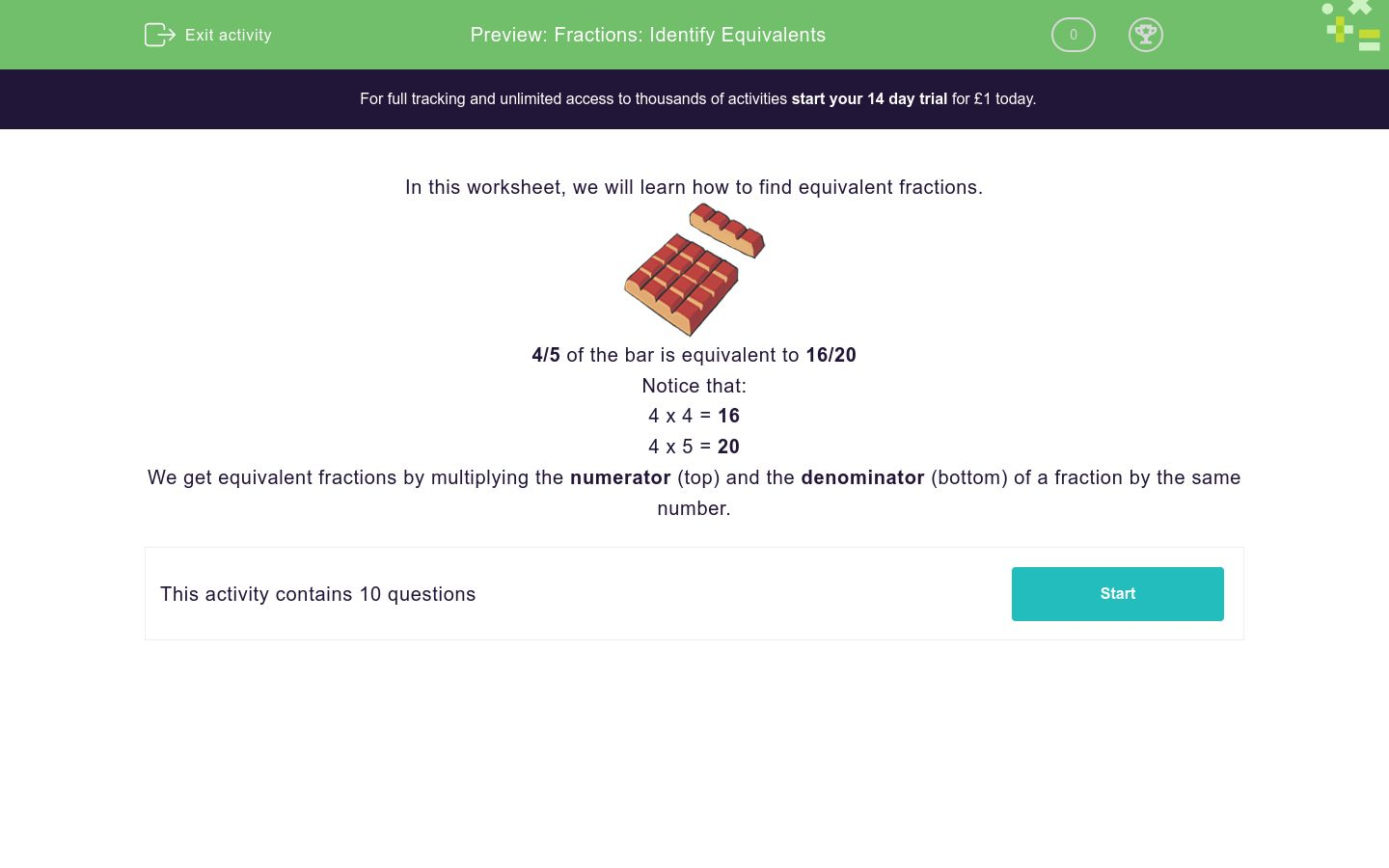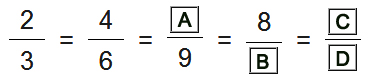# Fractions: Identify Equivalents

In this worksheet, students identify and write equivalent fractions for the given fraction.Key stage:  KS 2

Curriculum topic:  Number: Fractions, Decimals and Percentages

Curriculum subtopic:  Identify Equivalent Fractions

Difficulty level:### QUESTION 1 of 10

In this worksheet, we will learn how to find equivalent fractions.4/5 of the bar is equivalent to 16/20

Notice that:

4 x 4 = 16

4 x 5 = 20

We get equivalent fractions by multiplying the numerator (top) and the denominator (bottom) of a fraction by the same number

Find more equivalent fractions.## Column B

A
25
B
20
C
3
D
5

Find more equivalent fractions.## Column B

A
6
B
10
C
24
D
30

Find more equivalent fractions.## Column B

A
30
B
20
C
12
D
24

Find more equivalent fractions.## Column B

A
32
B
35
C
21
D
40

Find more equivalent fractions.## Column B

A
6
B
15
C
12
D
10

Find more equivalent fractions.## Column B

A
16
B
20
C
3
D
5

Find more equivalent fractions.## Column B

A
30
B
12
C
24
D
20

Find more equivalent fractions.## Column B

A
20
B
15
C
16
D
9

Find more equivalent fractions.## Column B

A
36
B
9
C
45
D
15

Find more equivalent fractions.## Column B

A
8
B
3
C
10
D
5
• Question 1

Find more equivalent fractions.## Column B

A
3
B
20
C
5
D
25
EDDIE SAYS
C and D follow the pattern - adding 1 to the numerator and 5 to the denominator
• Question 2

Find more equivalent fractions.## Column B

A
6
B
24
C
10
D
30
EDDIE SAYS
The pattern is adding 2 to the numerator and 6 to the denominator.
• Question 3

Find more equivalent fractions.## Column B

A
12
B
24
C
20
D
30
EDDIE SAYS
The pattern is adding 4 to the numerator and 6 to the denominator.
• Question 4

Find more equivalent fractions.## Column B

A
21
B
32
C
35
D
40
EDDIE SAYS
This time, the pattern is adding 7 to the numerator and 8 to the denominator.
• Question 5

Find more equivalent fractions.## Column B

A
6
B
12
C
10
D
15
EDDIE SAYS
The pattern adds 2 to the numerator and 3 to the denominator.
• Question 6

Find more equivalent fractions.## Column B

A
3
B
16
C
5
D
20
• Question 7

Find more equivalent fractions.## Column B

A
12
B
24
C
20
D
30
• Question 8

Find more equivalent fractions.## Column B

A
9
B
16
C
15
D
20
• Question 9

Find more equivalent fractions.## Column B

A
9
B
36
C
15
D
45
• Question 10

Find more equivalent fractions.## Column B

A
3
B
8
C
5
D
10
---- OR ----

Sign up for a £1 trial so you can track and measure your child's progress on this activity.

### What is EdPlace?

We're your National Curriculum aligned online education content provider helping each child succeed in English, maths and science from year 1 to GCSE. With an EdPlace account you’ll be able to track and measure progress, helping each child achieve their best. We build confidence and attainment by personalising each child’s learning at a level that suits them.

Start your £1 trial